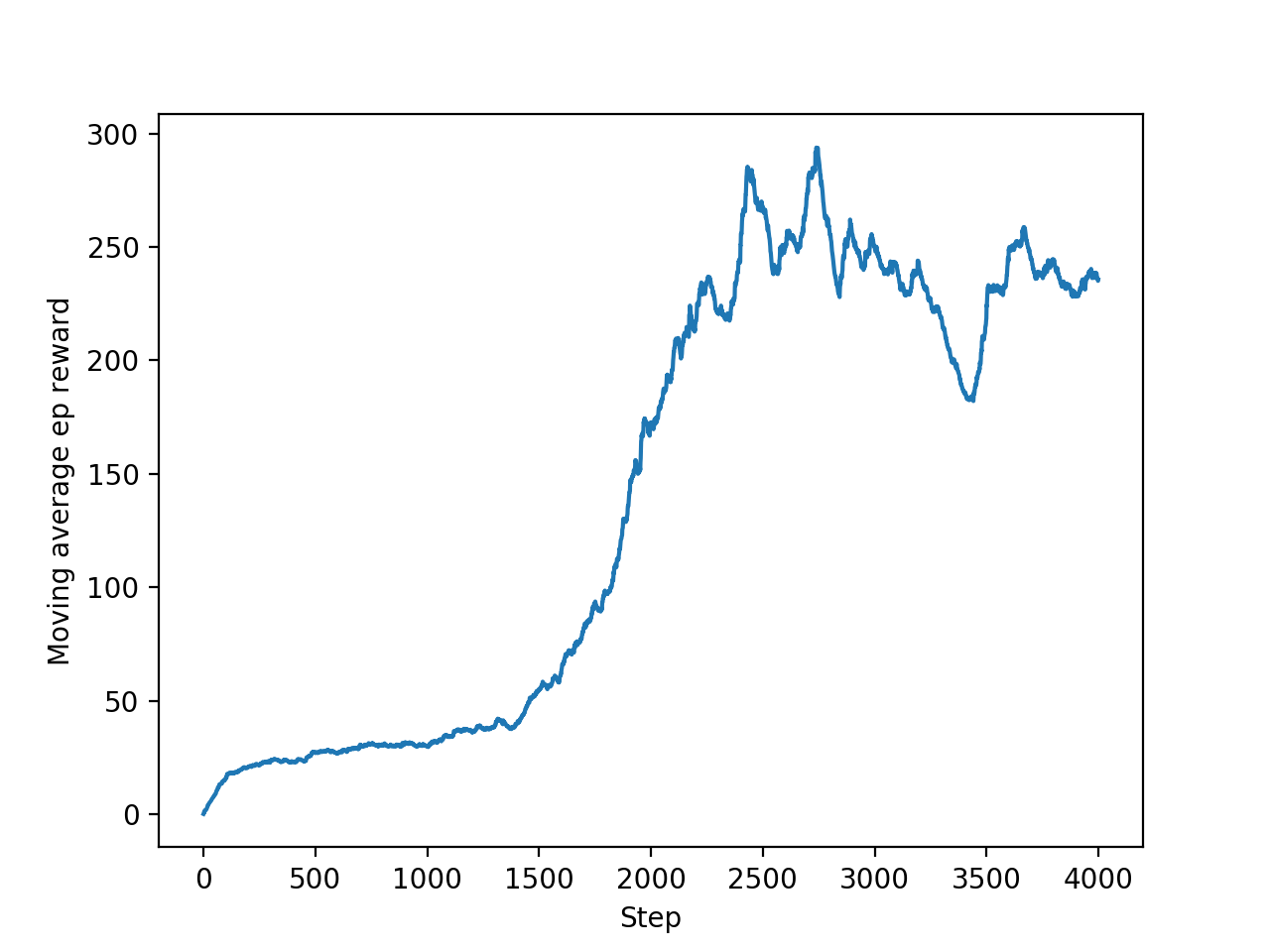# DQN 强化学习

## 要点 ¶

Torch 是神经网络库, 那么也可以拿来做强化学习, 之前我用另一个强大神经网络库 Tensorflow 来制作了这一个 从浅入深强化学习教程, 你同样也可以用 PyTorch 来实现, 这次我们就举 DQN 的例子, 我对比了我的 Tensorflow DQN 的代码, 发现 PyTorch 写的要简单很多. 如果对 DQN 或者强化学习还没有太多概念, 强烈推荐我的这个DQN动画短片, 让你秒懂DQN. 还有强推这套花了我几个月来制作的强化学习教程!

## 模块导入和参数设置 ¶

import torch
import torch.nn as nn
import torch.nn.functional as F
import numpy as np
import gym

# 超参数
BATCH_SIZE = 32
LR = 0.01                   # learning rate
EPSILON = 0.9               # 最优选择动作百分比
GAMMA = 0.9                 # 奖励递减参数
TARGET_REPLACE_ITER = 100   # Q 现实网络的更新频率
MEMORY_CAPACITY = 2000      # 记忆库大小
env = gym.make('CartPole-v0')   # 立杆子游戏
env = env.unwrapped
N_ACTIONS = env.action_space.n  # 杆子能做的动作
N_STATES = env.observation_space.shape   # 杆子能获取的环境信息数


## 神经网络 ¶

DQN 当中的神经网络模式, 我们将依据这个模式建立两个神经网络, 一个是现实网络 (Target Net), 一个是估计网络 (Eval Net).

class Net(nn.Module):
def __init__(self, ):
super(Net, self).__init__()
self.fc1 = nn.Linear(N_STATES, 10)
self.fc1.weight.data.normal_(0, 0.1)   # initialization
self.out = nn.Linear(10, N_ACTIONS)
self.out.weight.data.normal_(0, 0.1)   # initialization

def forward(self, x):
x = self.fc1(x)
x = F.relu(x)
actions_value = self.out(x)
return actions_value


## DQN体系 ¶

class DQN(object):
def __init__(self):
# 建立 target net 和 eval net 还有 memory

def choose_action(self, x):
# 根据环境观测值选择动作的机制
return action

def store_transition(self, s, a, r, s_):
# 存储记忆

def learn(self):
# target 网络更新
# 学习记忆库中的记忆


class DQN(object):
def __init__(self):
self.eval_net, self.target_net = Net(), Net()

self.learn_step_counter = 0     # 用于 target 更新计时
self.memory_counter = 0         # 记忆库记数
self.memory = np.zeros((MEMORY_CAPACITY, N_STATES * 2 + 2))     # 初始化记忆库
self.optimizer = torch.optim.Adam(self.eval_net.parameters(), lr=LR)    # torch 的优化器
self.loss_func = nn.MSELoss()   # 误差公式

def choose_action(self, x):
x = torch.unsqueeze(torch.FloatTensor(x), 0)
# 这里只输入一个 sample
if np.random.uniform() < EPSILON:   # 选最优动作
actions_value = self.eval_net.forward(x)
action = torch.max(actions_value, 1).data.numpy()[0, 0]     # return the argmax
else:   # 选随机动作
action = np.random.randint(0, N_ACTIONS)
return action

def store_transition(self, s, a, r, s_):
transition = np.hstack((s, [a, r], s_))
# 如果记忆库满了, 就覆盖老数据
index = self.memory_counter % MEMORY_CAPACITY
self.memory[index, :] = transition
self.memory_counter += 1

def learn(self):
# target net 参数更新
if self.learn_step_counter % TARGET_REPLACE_ITER == 0:
self.learn_step_counter += 1

# 抽取记忆库中的批数据
sample_index = np.random.choice(MEMORY_CAPACITY, BATCH_SIZE)
b_memory = self.memory[sample_index, :]
b_s = torch.FloatTensor(b_memory[:, :N_STATES])
b_a = torch.LongTensor(b_memory[:, N_STATES:N_STATES+1].astype(int))
b_r = torch.FloatTensor(b_memory[:, N_STATES+1:N_STATES+2])
b_s_ = torch.FloatTensor(b_memory[:, -N_STATES:])

# 针对做过的动作b_a, 来选 q_eval 的值, (q_eval 原本有所有动作的值)
q_eval = self.eval_net(b_s).gather(1, b_a)  # shape (batch, 1)
q_next = self.target_net(b_s_).detach()     # q_next 不进行反向传递误差, 所以 detach
q_target = b_r + GAMMA * q_next.max(1)   # shape (batch, 1)
loss = self.loss_func(q_eval, q_target)

# 计算, 更新 eval net
loss.backward()
self.optimizer.step()


## 训练 ¶

dqn = DQN() # 定义 DQN 系统

for i_episode in range(400):
s = env.reset()
while True:
env.render()    # 显示实验动画
a = dqn.choose_action(s)

# 选动作, 得到环境反馈
s_, r, done, info = env.step(a)

# 修改 reward, 使 DQN 快速学习
x, x_dot, theta, theta_dot = s_
r1 = (env.x_threshold - abs(x)) / env.x_threshold - 0.8
r = r1 + r2

# 存记忆
dqn.store_transition(s, a, r, s_)

if dqn.memory_counter > MEMORY_CAPACITY:
dqn.learn() # 记忆库满了就进行学习

if done:    # 如果回合结束, 进入下回合
break

s = s_


## 附加 A3C ¶